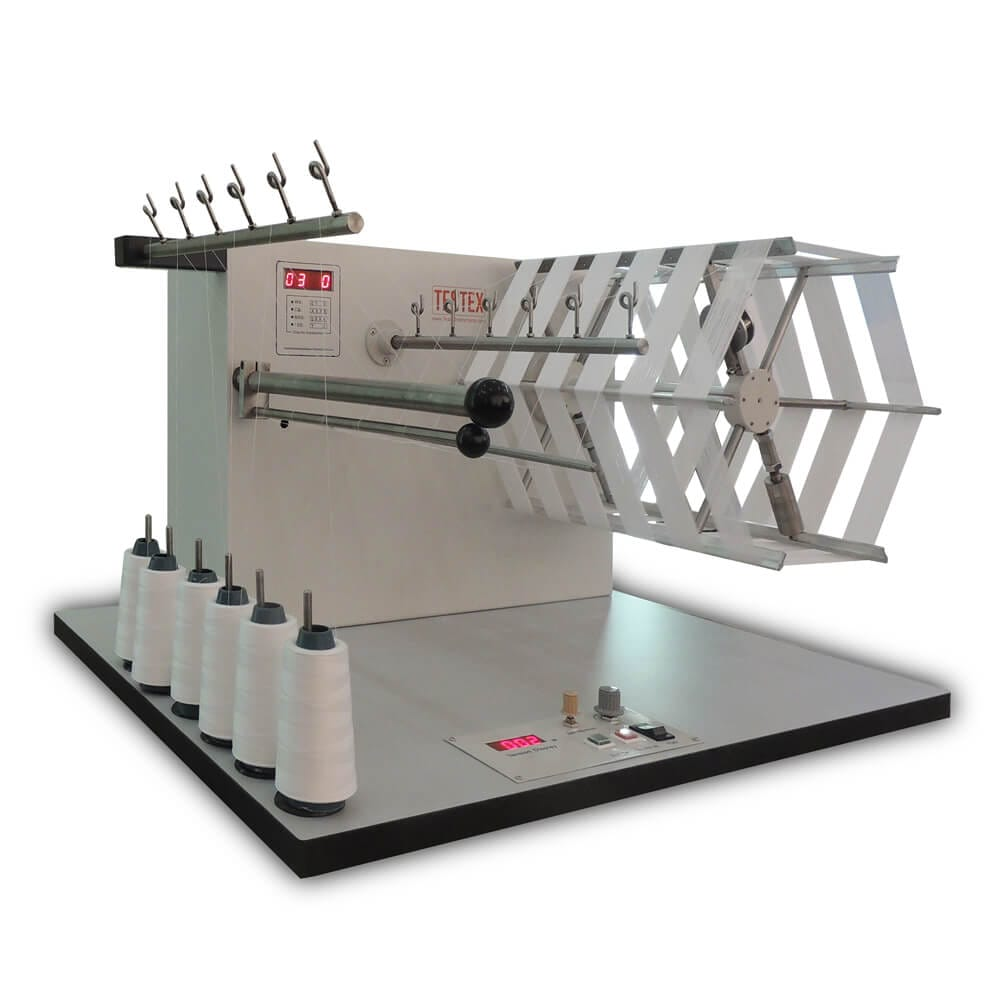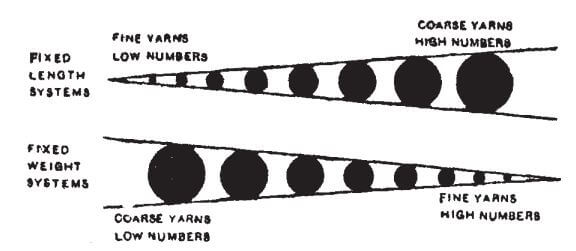# Yarn Count | Different Counting Systems

To become a successful textile personnel one must have the out-and-out knowledge on yarn count and different yarn counting systems. This is one of the first and foremost basics that one should possess to survive in the industry of textile. The best weaving loom and knitting machines require yarns of appropriate yarn count. By the end of this article on yarn count, you will have a clear concept on –

• What is the yarn count?
• What significance does it have in handling threads and yarns?
• What are the classifications of the yarn counting system?
• How is the conversion done among different counting systems?
• Which machines are used for measuring yarn count?

So, without any further ado, let’s get into the business and explore the world of yarn counts and it’s various counting systems.## What is Yarn Count?

Yarn count is nothing but an expression that leads one to a clear vision of the fineness and coarseness of threads and yarns. In other words, it signifies the size and thickness or thinness of a particular thread. Basically, count determines yarn’s weight in a unit length or sometimes yarn’s length in unit weight.## Importance of Yarn Count

• To differentiate among the finer and the coarser yarn
• To anticipate the thickness and thinness of the yarn
• To anticipate the weight of the unit length of the yarn
• To anticipate the length of the unit weight of the yarn
• To anticipate the gsm of the finished fabric
• To anticipate the linear density of the yarn

## Classification of Yarn Counting System

The yarn counting system can be classified into mainly two categories. Direct counting system and indirect counting system. In the direct counting system, the unit length of yarn is fixed and the weight is variable. On the other hand, in the indirect yarn counting system, the unit weight of thread is fixed and the length of thread is variable. The classification of yarn counting system is as follows:### Direct Counting System: (fixed unit length and variable weight)

A few examples of direct counting system is provided here-

#### Tex

Tex is the universal yarn counting system. In this system, the length of yarn is fixed and that is 1000 meter. The weight of 1000 meter yarn in grams is said to be the Tex count of a yarn. So, 20 Tex count implies that a yarn of 1000 meter weighs 20 grams.

Tex is used for mainly yarns that are composed of natural fibres. DeciTex (DTex), MiliTex (MTex) and KiloTex (KTex) are the derivatives of the Tex counting system.

#### Denier

Denier counting system is mainly used for yarns that are made from finer fibres. The weight of 9000 meter yarn in grams is said to be the Denier count of a yarn. So, 20 Denier count implies that a yarn of 9000 meter weighs 20 grams.

Denier is used for yarns comprising synthetic or man-made fibre or filament. Fibre below 1 Denier is said to be microfiber.

#### Jute Counting System (lbs/spyndle)

In this system, the fixed length is 14400 yards and the weight unit is in pounds. So, the weight of 14400 yards in pounds unit is said to be the Jute count of that yarn.

Jute, Flax, Hemp fibers are mainly handled in this counting system.

“In the direct counting system, with the increase of count value the yarn gets coarser or thicker and vice-versa.”### Indirect Counting System: (fixed unit weight and variable length)

A few examples of indirect counting system is provided here-

#### English Count

In this counting system, hanks of 840 yards are prepared at first. Then, the number of hanks required for weighing 1 pound of yarn is said to be the English Count of the yarn. It is denoted as Ne. This counting method is also known as the cotton counting system.

A 20 Ne yarn means, 20 hanks of 840 yards yarn weigh 1 pound or (20×840) yards = 16,800 yards yarn weigh 1 pound.

#### Metric Count

In this method, hanks are prepared of 1000 meter length. The number of hanks required for weighing 1 kilogram of yarn is said to be the Metric Count of the yarn. It is denoted as Nm.

A 20 Nm yarn means, 20 hanks of 1000 meter yarn weigh 1 kilogram or (20×1000) meter = 20,000 meter yarn weigh 1 kilogram.

#### Worsted Count

In this counting system, hanks of 560 yards are prepared at first. Then, the number of hanks required for weighing 1 pound of yarn is said to be the Worsted Count of the yarn. It is denoted as Nw. This counting method is also known as the Mohair counting system.

A 20 Nw yarn means, 20 hanks of 560 yards yarn weigh 1 pound or (20×560) yards = 11,200 yards yarn weigh 1 pound.

“In the indirect counting system, with the increase of count value the yarn gets finer or thinner and vice-versa.”## Count Conversation System

Yarn Counts can be converted in following manner:Yarn Count Conversion

For instance, 20 Tex = 180 Denier = 0.570 Jute Count =  29.5 Ne = 50 Nm = 44 Nw

## Count Measuring Machine

For measuring yarn count a wrap reel machine and a digital balance is needed. A lea (120 yards)  of yarn has been wrapped in a wrap reel machine. After that, it has been weighed in a digital balance and this sort of reading has been taken for several times. From the following formula, yarn count can be measured in English Count (Ne) or Cotton Count System:Yarn Count Testing Machine

Ne = (L/l) / (W/w)

L = length of sample

l  = length of reference length

W = weight of sample

w  = weight of reference lengthDigital Balance for Yarn Count Testing

This a semi automatic system for measuring yarn count. But, now there are a lot of automated machines available for measuring yarn count.

### 1. What is DTex and MTex?

These are derivatives of Tex. Here, DTex stands for decitex and MTex stands for militex. Weight of 1000 meter yarn in decigram or milligram is said to be DTex and MTex respectively.

### 2. “As the count increases yarns get finer.”  – Is this true?

No. Not always. In the indirect system, where the unit weight is fixed; here, as the count increases, yarn gets finer but in the direct system, where the unit length is fixed; here, as the count increases yarn gets thicker.Counting System

### 3. What is CN Tex?

This is actually the breaking length (expressed in km) of a yarn. It is that specific length of a yarn at which it breaks down due to its own weight. It is also known as RKM.

Tags:
0 replies